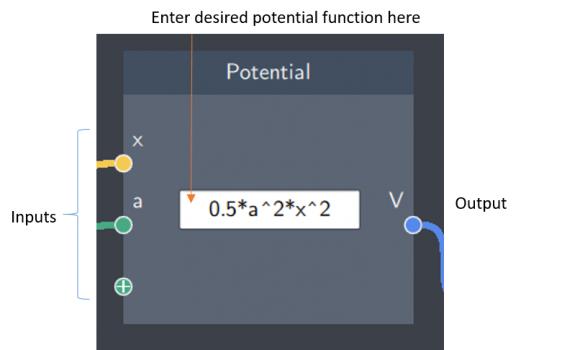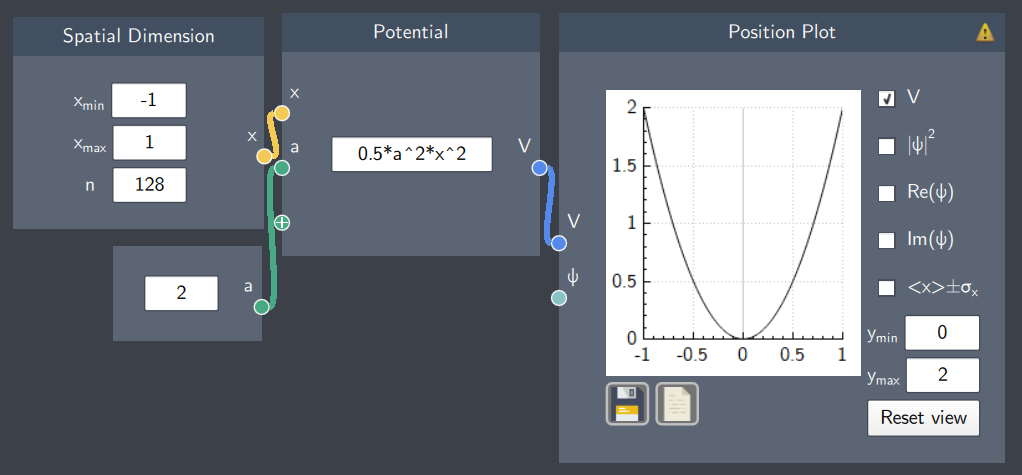# Potential

## Description

The Potential node lets you define the potential function of your system.## Input

The node has the following inputs:

• Spatial Dimension ($x$): It inputs the values of $x$-axis defined in the Spatial Dimension node.
• Scalars ($a$, $b$ etc.): It is possible to input scalar quantities to parameterize the expression.

## Content

The content field of the node consists of an analytical expression that defines the potential function of the system.

Note that several helpful functions are available, see https://www.quatomic.com/composer/reference/quantum-composer-basics/expressions/.

## Output

After all the inputs are provided, the node gives the following output:

• Potential ($V$) : A potential function defined by the written expression and its inputs.

## Example

An example is shown below where a harmonic oscillator potential is defined with the spatial dimensions and a scalar representing the frequency of the oscillator. The output of the potential function is shown on the plot.